# Calorimetry

• Define calorimetry.
• Perform calculations involving calorimetry relationships.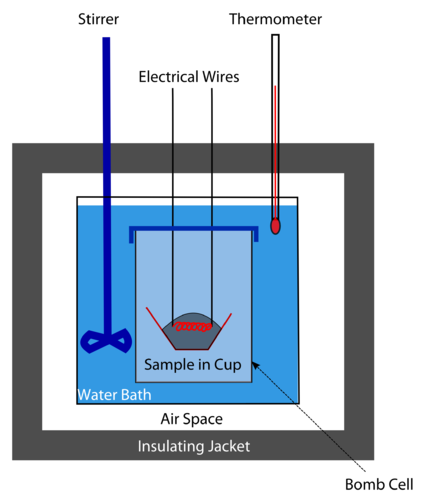How many calories are in your food?

At one time, calories in foods were measured with a bomb calorimeter (see figure above). A weighed amount of the food would be placed in the calorimeter and the system was then sealed and filled with oxygen. An electric spark ignited the food-oxygen mixture. The amount of heat released when the food burned would give an idea of the food calories present. Today calories are calculated from the protein, carbohydrate, and fat content of the food (all determined by chemical analysis). No more bombs needed.

### Calorimetry

Calorimetry is the measurement of the transfer of heat into or out of a system during a chemical reaction or physical process. A calorimeter is an insulated container that is used to measure heat changes. The majority of reactions that can be analyzed in a calorimetry experiment are either liquids or aqueous solutions. A frequently used and inexpensive calorimeter is a set of nested foam cups fitted with a lid to limit the heat exchange between the liquid in the cup and the air in the surroundings (see Figure below ). In a typical calorimetry experiment, specific volumes of the reactants are dispensed into separate containers and the temperature of each is measured. They are then mixed into the calorimeter, which starts the reaction. The reactant mixture is stirred until the reaction is complete, while the temperature of the reaction is continuously monitored.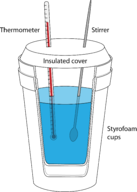Figure 17.6

A simple constant-pressure calorimeter.

The key to all calorimetry experiments is the assumption that there is no heat exchange between the insulated calorimeter and the room. Consider the case of a reaction taking place between aqueous reactants. The water in which the solids have been dissolved is the surroundings, while the dissolved substances are the system. The temperature change that is measured is the temperature change that is occurring in the surroundings. If the temperature of the water increases as the reaction occurs, the reaction is exothermic. Heat was released by the system into the surrounding water. An endothermic reaction absorbs heat from the surroundings, so the temperature of the water decreases as heat leaves the surroundings to enter the system.

The temperature change of the water is measured in the experiment and the specific heat of water can be used to calculate the heat absorbed by the surroundings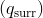.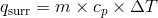In the equation,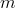is the mass of the water,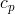is the specific heat of the water, and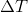is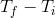. The heat absorbed by the surroundings is equal, but opposite in sign, to the heat released by the system. Because the heat change is determined at constant pressure, the heat released by the system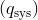is equal to the enthalpy change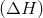.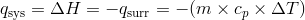The sign of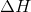is positive for an endothermic reaction and negative for an exothermic reaction.

#### Sample Problem: Calorimetry and Enthalpy Changes

In an experiment, 25.0 mL of 1.00 M HCl at 25.0°C is added to 25.0 mL of 1.00 M NaOH at 25.0°C in a foam cup calorimeter. A reaction occurs and the temperature rises to 32.0°C. Calculate the enthalpy changein kJ for this reaction. Assume the densities of the solutions are 1.00 g/mL and that their specific heat is the same as that of water.

Step 1: List the known quantities and plan the problem .

Known

•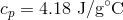•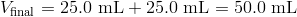•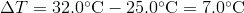• Density = 1.00 g/mL

Unknown

•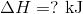The volume and density can be used to find the mass of the solution after mixing. Then calculate the change in enthalpy by using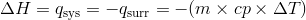.

Step 2: Solve .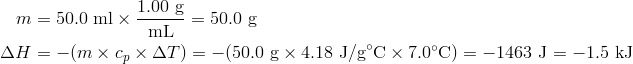Step 3: Think about the result .

The enthalpy change is negative because the reaction releases heat to the surroundings, resulting in an increase in temperature of the water.

#### Summary

• The process of calorimetry is described.
• Calculations involving enthalpy changes are illustrated.

#### Practice

Work the problems at the link below:

http://misterguch.brinkster.net/PRA047.pdf

#### Review

Questions

1. What kinds of reactions are usually analyzed in a calorimeter?
2. What is a constant-pressure calorimeter?
3. Why are foam cups used in a calorimeter?
• calorimeter: An insulated container that is used to measure heat changes.
• calorimetry: The measurement of the transfer of heat into or out of a system during a chemical reaction or physical process.